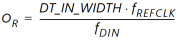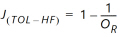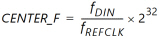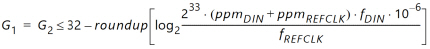# NIDRU Configuration

## Clock and Data Recovery Unit based on Deserialized Oversampled Data (XAPP1240)

Document ID
XAPP1240
Release Date
2022-11-04
Revision
3.1 English

This section describes how to translate the incoming data rate and reference clock frequency into a valid NIDRU configuration.

The user configuration defines specifications for:

Incoming data rate with associated tolerance (fDIN ± ppm)

Available reference clock frequency with associated tolerance (fREFCLK ± ppm)

While fDIN is given, fREFCLK can be selected inside a valid range. The range upper limit comes from the necessity to close timing in the target device, and is thus device and speed grade dependent. The lower limit to fREFCLK might be imposed by the PHY. For example, a SerDes typically specifies a minimum reference clock frequency. A SelectIO interface does not typically impose a lower limit to fREFCLK.

The maximum fDIN is typically limited by the oversampling rate OR defined in This Equation:

Equation 2Although the OR has to be at least > 2, Xilinx recommends to keep OR ≥ 3 to have enough high frequency jitter tolerance.

The theoretical high frequency jitter tolerance is related to OR as defined in This Equation:

Equation 3All DRUs for which This Equation is valid have an optimal J(TOL-HF).

Use This Equation and This Equation to calculate the NIDRU parameters CENTER_F, G1, and G2. This Equation calculates CENTER_F:

Equation 4This Equation calculates G1 and G2:

Equation 5The spreadsheet nidru_transfer_function_v_1_0.xls in the reference design folder /excel_plots implements the equations listed above.

Using an equal value for G1 and G2 guarantees that the NIDRU operates in the lock-in region over the full tolerance range (ppm) of both the incoming data and the reference clock. Further reducing G2 increases the NIDRU bandwidth. Increasing G2 is not recommended because the NIDRU would operate in the pull-in region where the automatic lock is not always guaranteed.

G1_P can be evaluated using the spreadsheet nidru_transfer_function_v_1_0.xls in the reference design folder /excel_plots. The G1_P value should be increased until the ringing effect on the output phase becomes negligible. When G1 = G2, setting G1_P = 16 guarantees a negligible ringing effect. Thus, G1_P = 16 is good for most cases.